Most Affordable JEE | NEET | 8,9,10 Preparation by Kota's Top IITian Doctor Faculties

# RD Sharma Solutions for Class 9 Maths Chapter 11 Coordinate Geometry - Free PDF Download

Class 9Hey, are you a class 9 student and looking for ways to download RD Sharma Solutions for Class 9 Maths Chapter 11 "Coordinate Geometry"? If yes. Then read this post till the end.

In this article, we have listed RD Sharma Solutions for Class 9 Maths Chapter 11 in PDF that is prepared by Kota’s top IITian’s Faculties by keeping Simplicity in mind.

If you want to learn and understand class 9 Maths Chapter 11 "Coordinate Geometry" in an easy way then you can use these solutions PDF.

Chapter 11 of RD Sharma Class 9 deals with coordinate geometry and concepts such as the cartesian plane, coordinates of a point, names and terms associated with the coordinate plane, notations, plotting points in the plane and more. If you want to improve your basic in Coordinate Geometry, then you can use this.

RD Sharma Solutions helps students to Practice important concepts of subjects easily. RD Sharma class 9 solutions provide detailed explanations of all the  exercise questions that students can use to clear their doubts instantly.

If you want to become good at Math then it is very important for you to have a good knowledge of all the important topics of class 9 math, so to learn and practice those topics you can use eSaral RD Sharma Solutions.

So, without wasting more time Let’s start.

## RD Sharma Solutions for Class 9 Maths Chapter 11 Coordinate Geometry - Free PDF Download

Question 1: Plot the following points on the graph paper:

(i) $(2,5)$

(ii) $(4,-3)$

(iii) $(-5,-7)$

(iv) $(7,-4)$

(v) $(-3,2)$

(vi) $(7,0)$

(vii) $(-4,0)$

(viii) $(0,7)$

(ix) $(0,-4)$

(x)$(0,0)$

Solution: The following points are given below.

(i) $(2,5)$

(ii) $(4,-3)$

(iii) $(-5,-7)$

(iv) $(7,-4)$

(v) $(-3,2)$

(vi) $(7,0)$

(vii) $(-4,0)$

(viii) $(0,7)$

(ix) $(0,-4)$

(x)$(0,0)$

Let $X^{\prime} O X$ and $Y^{\prime} O Y$ be the coordinate axes,

(i) Here for the given point the abscissa is 2 units and ordinate is 5 units.

The point is in the first quadrant. So it will look like as shown in the following figure.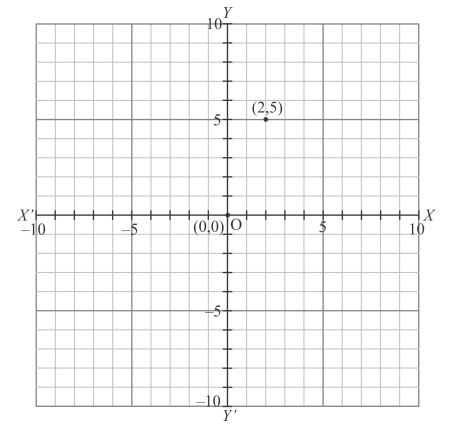(ii) Here for the given point the abscissa is 4 units and ordinate is $-3$ units.

The point is in the fourth quadrant. So it will look like as shown in the following figure.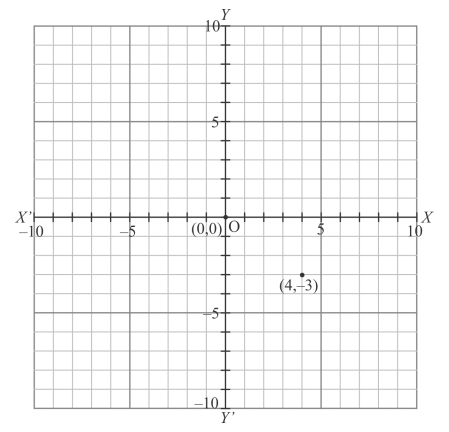(iii) Here for the given point the abscissa is $-5$ units and ordinate is $-7$ units.

The point is in the third quadrant. So it will look like as shown in the following figure.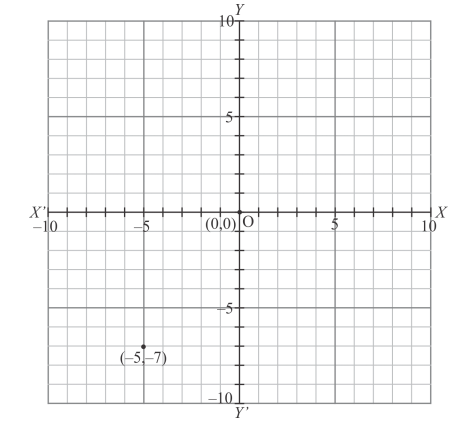(iv) Here for the given point the abscissa is 7 units and ordinate is $-4$ units.

The point is in the fourth quadrant. So it will look like as shown in the following figure.(v) Here for the given point the abscissa is $-3$ units and ordinate is 2 units.

The point is in the second quadrant. So it will look like as shown in the following figure.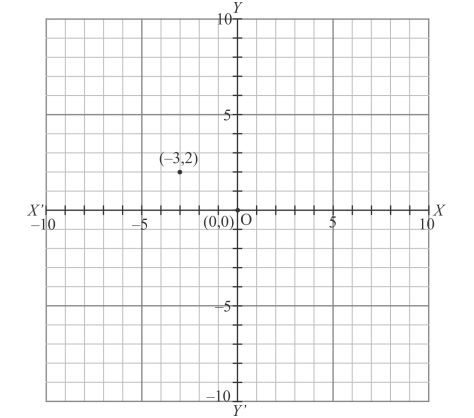(vi) Here for the given point the abscissa is 7 units and ordinate is 0 units.

The point is on the $x$-axis. So it will look like as shown in the following figure.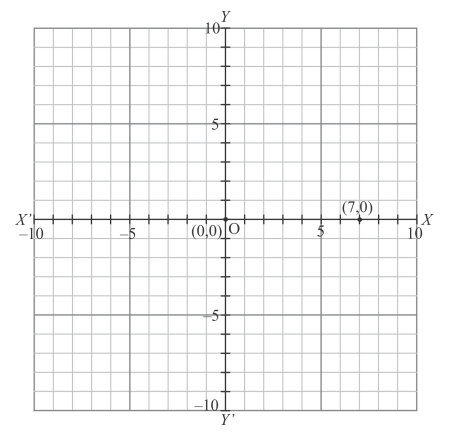(vii) Here for the given point the abscissa is $-4$ units and ordinate is 0 units.

The point is on the $x$-axis. So it will look like as shown in the following figure.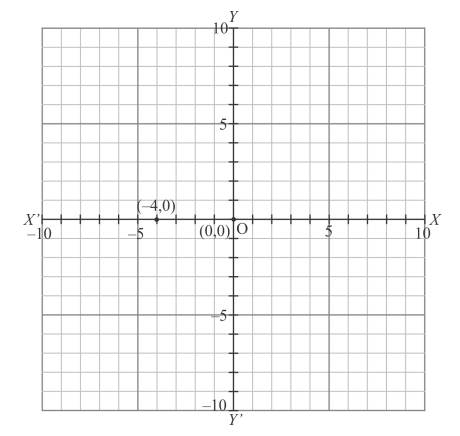(viii) Here for the given point the abscissa is 0 units and ordinate is 7 units.

The point is on the $y$-axis. So it will look like as shown in the following figure.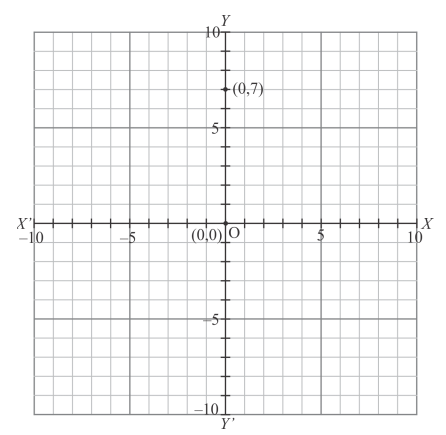(ix) Here for the given point the abscissa is 0 units and ordinate is $-4$ units.

The point is on the $y$-axis. So it will look like as shown in the following figure.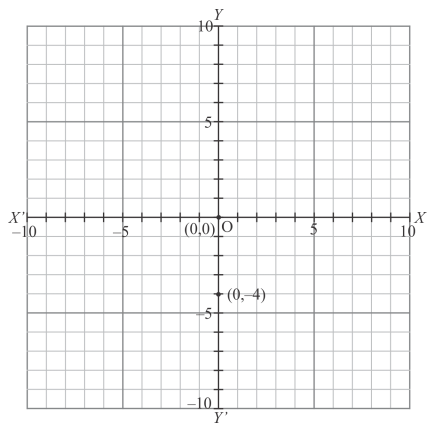(x) Here for the given point the abscissa is 0 units and ordinate is 0 units.

The point is basically intersection of the coordinate axes. So it will look like as shown in the following figure.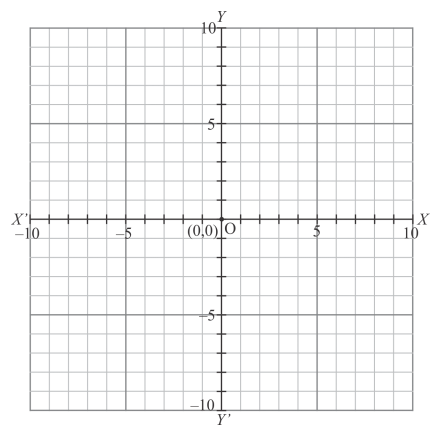Question 2: Write the coordinates of each of the following points marked in the graph paper: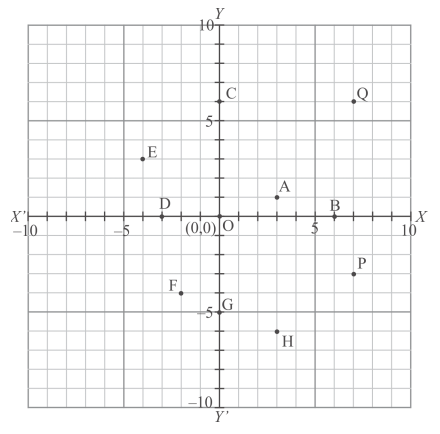Solution: The following graph is given in the question with the marked points and we are asked to write down their coordinates.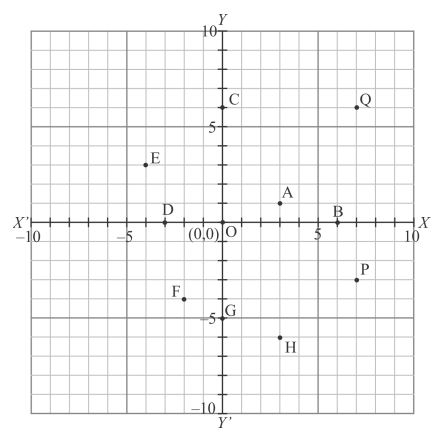The distance of point A from y-axis is 3 units and that of from x-axis is 1 units. Since A lies in the first quadrant, so its coordinates are .

The distance of point B from y-axis is 6 units and that of from x-axis is 0 units. Since B lies on x-axis, so its coordinates are .

The distance of point C from y-axis is 0 units and that of from x-axis is 6 units. Since C lies on y-axis, so its coordinates are .

The distance of point D from y-axis is -3 units and that of from x-axis is 0 units. Since D lies on x-axis, so its coordinates are .

The distance of point E from y-axis is -4 units and that of from x-axis is 3 units. Since E lies in the second quadrant, so its coordinates are .

The distance of point F from y-axis is -2 units and that of from x-axis is -4 units. Since F lies in the third quadrant, so its coordinates are .

The distance of point G from y-axis is 0 units and that of from x-axis is -5 units. Since G lies on y-axis, so its coordinates are .

The distance of point H from y-axis is 3 units and that of from x-axis is -6 units. Since H lies in the fourth quadrant, so its coordinates are .

The distance of point P from y-axis is 7 units and that of from x-axis is -3 units. Since P lies in the fourth quadrant, so its coordinates are .

The distance of point Q from y-axis is 7 units and that of from x-axis is 6 units. Since Q lies in the first quadrant, so its coordinates are

Question 3: Mark the correct alternative in each of the following:

The point of intersect of the coordinate axes is

(a) ordinate

(b) abscissa

(d) origin

Solution: As we know that:

The distance of a point from y−axis is called its x−coordinate or abscissa.

The distance of a point from x−axis is called its y−coordinate or ordinate.

The coordinate axes divide the plane into four equal parts which are known as quadrants.

The point of intersection of the coordinate axes is called the origin and the coordinates of origin are (0,0)

Example is shown in the graph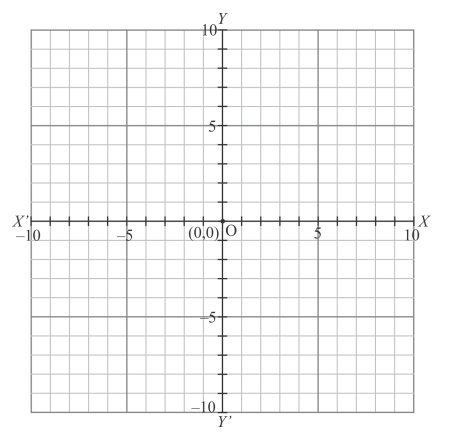Thus the correct answer is (d).

Question 4: The abscissa and ordinate of the origin are

(a) $(0,0)$

(b) $(1,0)$

(c) $(0,1)$

(d) $(1,1)$

Solution: As we know that:

The distance of a point from y−axis is called its x−coordinate or abscissa.

The distance of a point from x−axis is called its y−coordinate or ordinate.

The coordinate axes divide the plane into four equal parts which are known as quadrants.

The point of intersection of the coordinate axes is called the origin and the coordinates of origin are(0,0)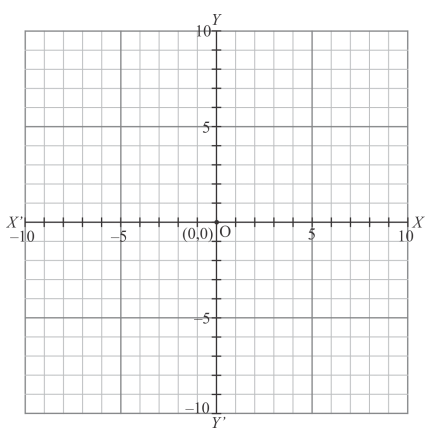The origin is shown in the graph

Thus the correct answer is (a).

Question 5: The measure of the angle between the coordinate axes is

(a) 0°

(b) 90°

(c) 180°

(d) 360°

Solution: As we know that $x$-axis and $y$-axis intersect to each other at point $\mathrm{O}$ and perpendicular to each other. So, the angle between the coordinate axes is $90^{\circ}$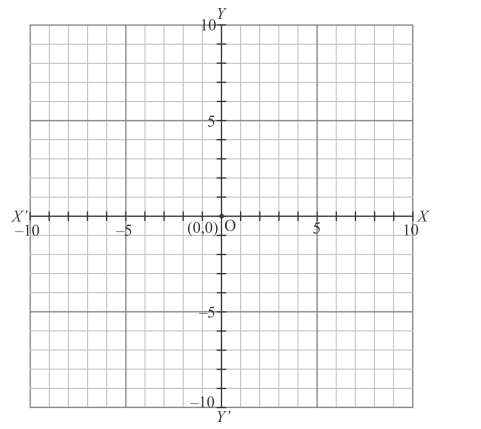Thus the correct answer is (b).

Question 6: A point whose abscissa and ordinate are 2 and −5 respectively, lies in

Solution: As shown in graph that a point whose abscissa and ordinate are 2 and $-5$ respectively lies in the fourth quadrant.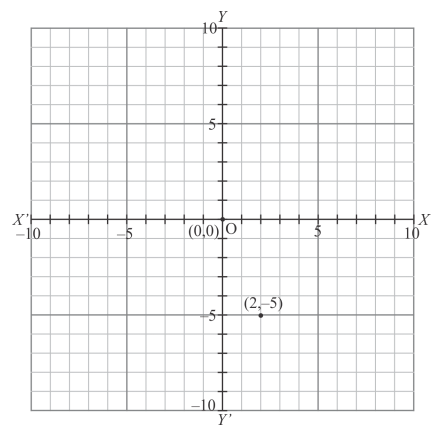Thus the correct answer is (d).

Question 7: Points (−4, 0) and (7, 0) lie

(a) on x-axis

(b) y-axis

Solution: Let the points $P$ and $Q$ whose coordinates are $(-4,0)$ and $(7,0)$ respectively. Locate the points and you will see that they lie on $x$-axis.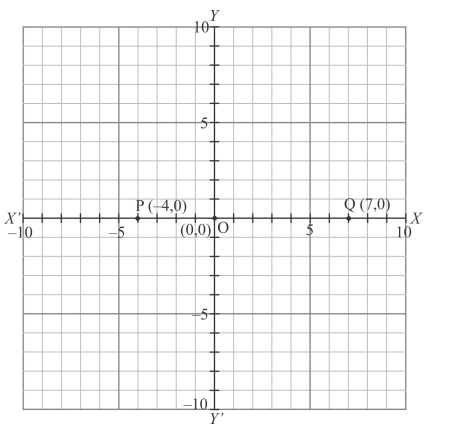Thus the correct answer is (a).

Question 8: The ordinate of any point on x-axis is

(a) 0

(b) 1

(c) −1

(d) any number

Solution: We know that the $y$-coordinates of every point on $x$-axis are zero. So, the coordinates of any point on the $x$-axis are of the form $(x, 0)$.

Thus the correct answer is (a).

Question 9: The abscissa of any point on y-axis is

(a) 0

(b) 1

(c) −1

(d) any number

Solution: We know that the $x-$ coordinate of every point on $y$-axis is zero. So, the coordinates of any point on the $x-$ axis are of the form $(0, y)$.

Thus the correct answer is (a).

Question 10 : The abscissa of a point is positive in the

Solution: The signs of coordinates $(x, y)$ of a point in various quadrants are shown in the following graph: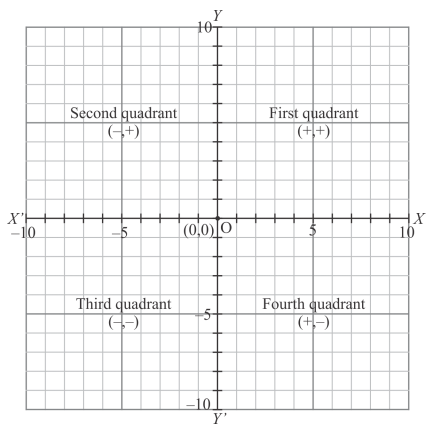Thus the correct answer is (d).

Question 11 : A point whose abscissa is $-3$ and ordinate 2 lies in

Solution: As we know that

In the first quadrant : $x>0, y>0$

In the second quadrant $: x<0, y>0$

In the third quadrant : $x<0, y<0$

In the fourth quadrant : $x>0, y<0$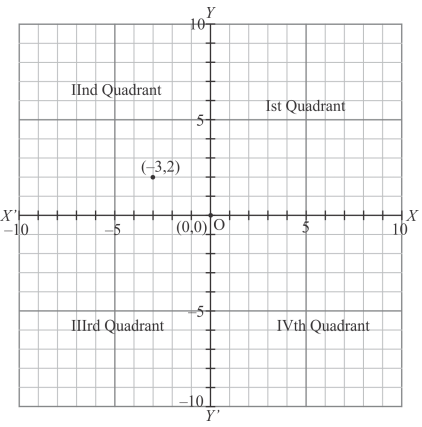The point whose abscissa is $-3$ which is negative and ordinate 2 is positive, so this point lies in the second quadrant.

Thus the correct answer is (b).

Question 12: Two points having same abscissae but different ordinate lie on

(a) x-axis

(b) y-axis

(c) a line parallel to $y$-axis

(d) a line parallel to $x$-axis

Solution: Let the points $\mathrm{P}(3,5)$ and $\mathrm{Q}(3,2)$ having the same abscissa but different ordinates be shown in the graph given below: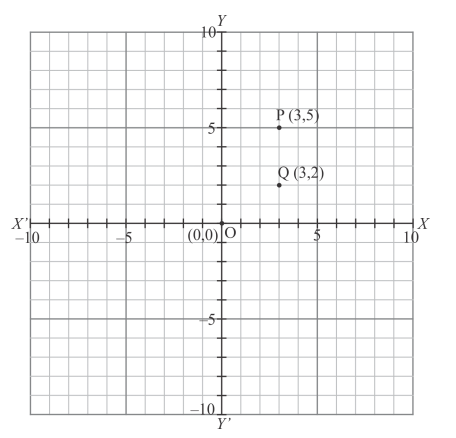Fig: (location of two considered points)

And these points lie on a line parallel to $y$-axis

Thus the correct answer is (c).

Question 13: The perpendicular distance of the point $P(4,3)$ from $x$-axis is

(a) 4

(b) 3

(c) 5

(d) none of these

Solution: The point $\mathrm{P}(4,3)$ is shown in the graph given below: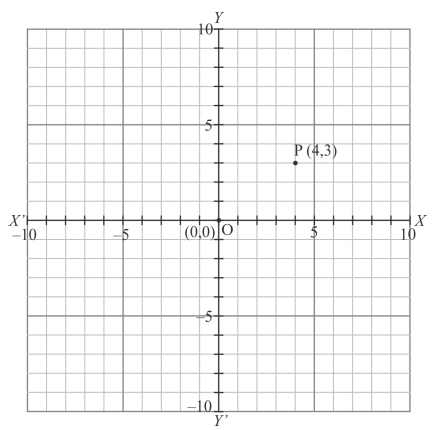Thus the perpendicular distance of the point $\mathrm{P}(4,3)$ from $x$-axis is 3 units.

Thus the correct answer is (b).

Question 14: The perpendicular distance of the $P(4,3)$ from $y$-axis is

(a) 4

(b) 3

(c) 5

(d) none of these

Solution: The point $\mathrm{P}(4,3)$ is shown in the graph given below: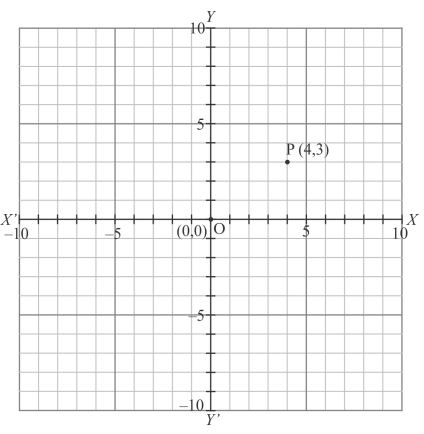Thus the perpendicular distance of the point $\mathrm{P}(4,3)$ from $y$-axis is 4 .

Thus the correct answer is (a).

Question 15: The distance of the point $P(4,3)$ from the origin is

(a) 4

(b) 3

(c) 5

(d) 7

Solution: The point $\mathrm{P}(4,3)$ is shown in the graph given below: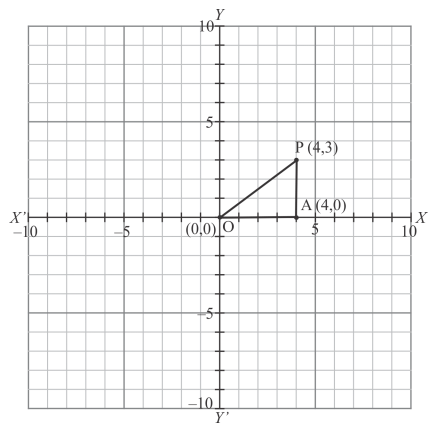In $\Delta \mathrm{OAP}$ is right angled triangle where

$\mathrm{OA}=4$

and $\mathrm{AP}=3$

By using Pythagoras theorem:

$\mathrm{OP}=\sqrt{\mathrm{OA}^{2}+\mathrm{AP}^{2}}$

$=\sqrt{4^{2}+3^{2}}$

$=\sqrt{16+9}$

$=\sqrt{25}$

$=5$

Thus the distance of the point $\mathrm{P}(4,3)$ from the origin is 5 .

Thus the correct answer is (c)

Question 16: The area of the triangle formed by the points $A(2,0) B(6,0)$ and $C(4,6)$ is

(a) 24 sq. units

(b) $12 \mathrm{sq}$. units

(c) 10 sq. units

(d) none of these

Solution: Given that points $\mathrm{A}(2,0), \mathrm{B}(6,0)$ and $\mathrm{C}(4,6)$ form a triangle which is shown in the figure. We are asked to find the area of the triangle $\triangle \mathrm{ABC} .$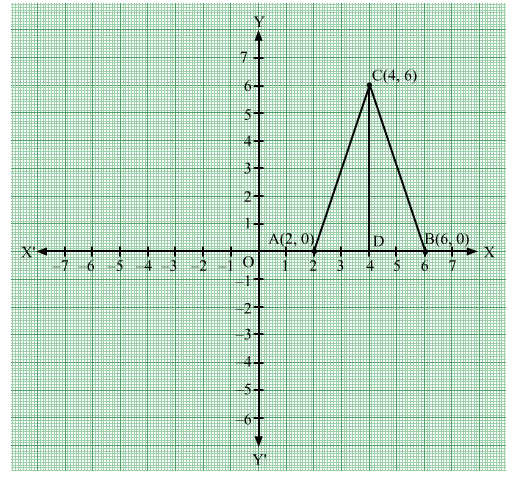Given that

$\mathrm{OA}=2$

and $\mathrm{OB}=6$

Hence:

$A B=O B-O A$

$=6-2$

$=4$

$C D=6$

By using formula,

$\Delta A B C=\frac{1}{2} \times A B \times C D$

$=\frac{1}{2} \times 4 \times 6$

$=12$ squnits

Thus the correct answer is (b).

Question 17: The area of the triangle formed by the points $P(0,1), Q(0,5)$ and $R(3,4)$ is

(a) $16 \mathrm{sq}$. units

(b) 8 sq. units

(c) 4 sq. units

(d) 6 sq. units

Solution: Given that the points $\mathrm{P}(0,1), \mathrm{Q}(0,5)$ and $\mathrm{R}(3,4)$ form a triangle.

We are asked to find the area of the triangle $\triangle \mathrm{PQR}$ which is shown in the figure.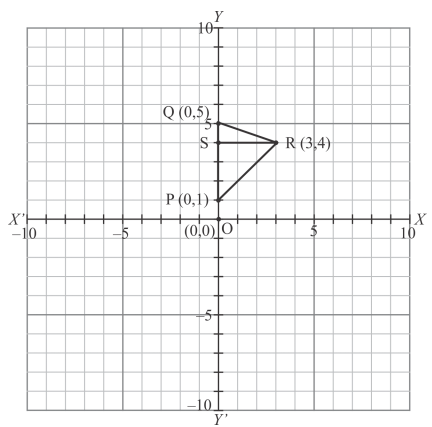Given that

$\mathrm{OP}=1$

and $\mathrm{OQ}=5$

Hence:

$\mathrm{PQ}=\mathrm{OQ}-\mathrm{OP}$

$=5-1$

$=4$

and $R S=3$

By using formula,

$\mathrm{PQR}=\frac{1}{2} \times \mathrm{PQ} \times \mathrm{RS}$

$=\frac{1}{2} \times 4 \times 3$

$=2 \times 3$

$=6 \mathrm{sq}$. Units

Thus the correct answer is $(\mathrm{d})$.